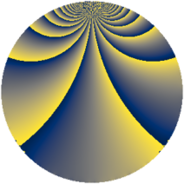Properties

 Label 1089.3.pLevel $1089$ Weight $3$ Character orbit 1089.p Rep. character $\chi_{1089}(10,\cdot)$ Character field $\Q(\zeta_{22})$ Dimension $1090$ Sturm bound $396$

Related objects

Defining parameters

 Level: $$N$$ $$=$$ $$1089 = 3^{2} \cdot 11^{2}$$ Weight: $$k$$ $$=$$ $$3$$ Character orbit: $$[\chi]$$ $$=$$ 1089.p (of order $$22$$ and degree $$10$$) Character conductor: $$\operatorname{cond}(\chi)$$ $$=$$ $$121$$ Character field: $$\Q(\zeta_{22})$$ Sturm bound: $$396$$

Dimensions

The following table gives the dimensions of various subspaces of $$M_{3}(1089, [\chi])$$.

Total New Old
Modular forms 2680 1110 1570
Cusp forms 2600 1090 1510
Eisenstein series 80 20 60

Trace form

 $$1090 q + 11 q^{2} + 201 q^{4} + 11 q^{5} - 11 q^{7} + 11 q^{8} + O(q^{10})$$ $$1090 q + 11 q^{2} + 201 q^{4} + 11 q^{5} - 11 q^{7} + 11 q^{8} - 99 q^{10} - 35 q^{11} - 17 q^{14} - 375 q^{16} + 11 q^{17} - 11 q^{19} - 41 q^{20} - 27 q^{22} + 117 q^{23} - 580 q^{25} + 195 q^{26} - 11 q^{28} + 11 q^{29} - 33 q^{31} + 11 q^{32} - 87 q^{34} + 11 q^{35} - 60 q^{37} + 241 q^{38} + 11 q^{41} - 11 q^{43} - 14 q^{44} - 11 q^{46} + 95 q^{47} + 978 q^{49} + 1155 q^{50} + 275 q^{52} - 357 q^{53} + 458 q^{55} - 530 q^{56} - 14 q^{58} + 161 q^{59} - 11 q^{61} + 616 q^{62} + 917 q^{64} - 220 q^{65} + 158 q^{67} + 11 q^{68} - 827 q^{70} - 17 q^{71} - 297 q^{73} + 11 q^{74} + 1210 q^{76} - 961 q^{77} - 1023 q^{79} - 1288 q^{80} - 389 q^{82} + 11 q^{83} + 55 q^{85} - 143 q^{86} + 1081 q^{88} - 338 q^{89} + 340 q^{91} - 1305 q^{92} + 275 q^{95} - 58 q^{97} + 1683 q^{98} + O(q^{100})$$

Decomposition of $$S_{3}^{\mathrm{new}}(1089, [\chi])$$ into newform subspaces

The newforms in this space have not yet been added to the LMFDB.

Decomposition of $$S_{3}^{\mathrm{old}}(1089, [\chi])$$ into lower level spaces

$$S_{3}^{\mathrm{old}}(1089, [\chi]) \cong$$ $$S_{3}^{\mathrm{new}}(121, [\chi])$$$$^{\oplus 3}$$$$\oplus$$$$S_{3}^{\mathrm{new}}(363, [\chi])$$$$^{\oplus 2}$$